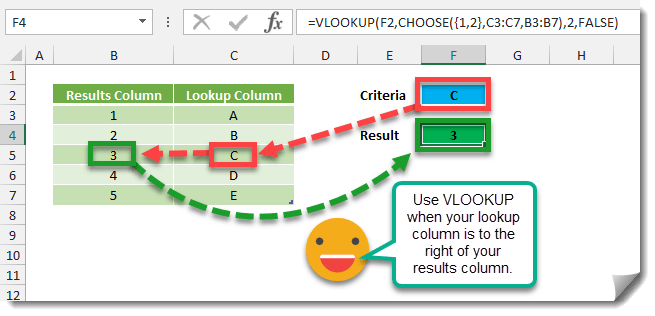VLOOKUP is one of the most useful functions in Excel, but after learning its use most people quickly realize some of its limitations.

• It only allows you to look up items to the right of a column with the criteria you’re looking up.
• It requires you to know how many columns away the results column is from the lookup column. This means if you insert a column later on your formula will break.

How can you get around these limitations and use VLOOKUP in any situation whether the results column is to the right or left and without worrying about adding columns and breaking the formula later on?

We can use the CHOOSE function with the VLOOKUP function.In this example the formula is looking up a value in Lookup Column and gives a result in Results Column. The CHOOSE function references each column individually and then creates a two column range for the the VLOOKUP. This avoids the issue of a changing column index number when a new column is inserted as the index number will always be 2.

``=VLOOKUP(F2,CHOOSE({1,2},C3:C7,B3:B7),2,FALSE)``